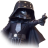# Correlation Percentile

S

#### Samus Aran

##### New member
hey everyone, I'm trying to make my own Correlation indicator with just adding as a modification the Mean & Percentile from the original indicator that ThinkorSwim provided . I got the mean to work, but I'm having trouble with the Percentile. I don't want the Percentile to be display on the graph; I want it display on the title of the indicator above its window, so I can get just coefficient Percentile of this instrument and compare the Percentile coefficient with other instruments.

sincerely

Code:
``````declare lower;

input length = 2000;
input correlationWithSecurity = "SPY";

plot Correlation = Correlation(close, close(correlationWithSecurity), length);
Correlation.SetDefaultColor(GetColor(5));

plot ratioAvg = ExpAverage(Correlation, length);

plot center = 0;

def currentCorrelation = Correlation(BarNumber(), close, length);

CorrelationPercentile = AsPercent((currentCorrelation / length));``````

S

#### Samus Aran

##### New member
nevermind the code above, let me reword my question: I would like to make a counter to count how many bars were under 0 and how many bars were above 0 on the Correlation axis of 0. And then compare the two percentages of above 0 & below 0 to see if these two instruments compared with each other are "for example 60%" negatively correlated or they are more positively correlated. Again I don't want it to be 60%, its just an example; I just want to find if most of the time if they are positively correlated or negatively correlated & what's that specific percentage.

Ty for the ones who decide to help me

Code:
``````declare lower;

input length = 14;
input length2 = 11682;

input correlationWithSecurity = "SPY";

plot Correlation =  Correlation(close, close(correlationWithSecurity), length);
Correlation.SetDefaultColor(GetColor(5));

plot ratioAvg = ExpAverage(Correlation, length);

plot center = 0;

rec postive = if Correlation > 0 then postive + 1 else 0;

rec negative = if Correlation < 0 then negative + 1 else 0;

def temp = Max ( postive,  negative);

plot PercentChg = 100 * ( temp / temp[length2] - 1);``````

Last edited:
G

#### generic

##### Member
@Samus Aran I don't really understand how counting the amount of bars above 0 will lead to correlation between 2 tickers. The Correlation study itself will tell you that from -1 to 1/ -100% to 100% based on some given length. So if length is 14 and study says .41 then correlation is 41% for the past 14 bars. Maybe I'm not getting it.

S

#### Samus Aran

##### New memberSPY signal based off of VIX correlation? Questions 5Correlation Indicator "Badge" ? Questions 5